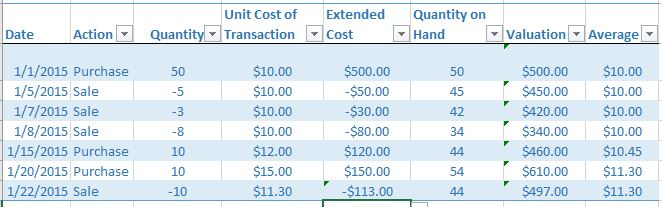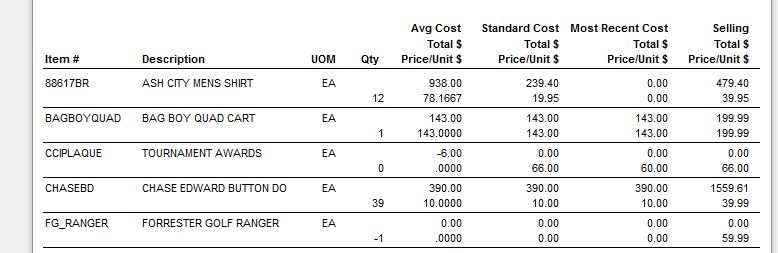# How do I read the Inventory Valuation report?

The  Inventory Valuation is a date sensitive report designed to tell you the value of your inventory at a point in time.

The table in blue below is a sample of various transactions.

On 1/1/15 the club purchased 50 units of an item at \$10.00 a unit, the overall cost is \$500.00.

Between January 5th - January 8th, 16 units were sold at the average cost of \$10.00.  Note, costing has nothing to do with the sale price.  This left the club with 34 units at \$10.00 per unit with an valuation of \$340.00  (34 X 10)

On January 15 the club purchased 10 more units at \$12.00 each.  This will raise the valuation and the unit cost.  We now have 44 units at a total cost/valuation of \$460.00 - the average cost is \$10.45.

On January 20 the club purchased 10 more units at \$15.00 each.  This will raise the valuation and the unit cost.  We now have 54 units at a total cost/valuation of \$610.00 - the average cost is \$11.30.

On January 22nd we had a sale of 10 units, the cost at this time is \$11.30.  Sales do NOT change the unit cost, only the quantity on hand.  Therefore our quantity fell back to 44, but our unit cost remains at \$11.30Below is a sample valuation report.

The Average Cost is based on actual numbers and calculations.   This is the number the accountants would use is their general ledger.

The Standard Cost is based on the costing number entered in the item profile.  In a perfect world, this would be the expected cost of the item times the quantity on hand.

The Most Recent Cost is based on the last purchase times the quantity on hand.

The Selling total is based on the sales price of the item times the quantity on hand.